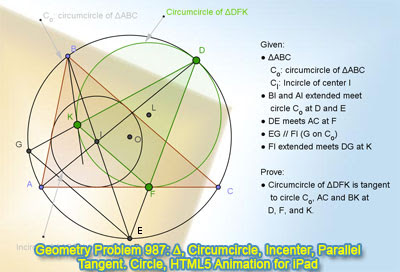## Saturday, February 22, 2014

### Geometry Problem 987: Triangle, Circumcircle, Incenter, Chord, Parallel, Circle, Tangent

Geometry Problem. GeoGebra, HTML5 Animation for iPad and Nexus.
Post your solution in the comments box below.

Level: Mathematics Education, High School, Honors Geometry, College.

Click the figure below to see the dynamic geometry demonstration of problem 987.#### 1 comment:

1.BC and AD meet at P. Because <DAC=<BAD=<PCD, triangles DAC and DCP are similar. DF bisects <ADC and BE bisects <ABC so AF/FC=AD/DC=DC/DP=AB/BP=AI/IP, making IF parallel to BC as well as the given GE.
Third parallel line allows us to say that <GEB=<B/2=<GDB, so <KDF=180-<A-<B/2-<B/2=<C=<KFA making AC tangent to circle L.
<KFD=<GED=<B/2+<A/2=<BID, but because <EBD=<DGE=<DKI makes BKID cyclic, <KFD=<BID=<BKD which also makes BK tangent to circle L.